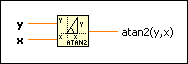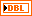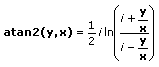# Inverse Tangent (2 Input) Function

## LabVIEW 2018 Help

Edition Date: March 2018
Part Number: 371361R-01
View Product Info

### DOWNLOAD (Windows Only)LabVIEW 2016 HelpLabVIEW 2017 HelpLabVIEW 2018 HelpLabVIEW 2019 HelpLabVIEW 2020 Help

Owning Palette: Trigonometric Functions

Requires: Base Development System

Computes the arctangent of y/x.

This function can compute the arctangent for angles in any of the four quadrants of the x-y plane, whereas the Inverse Tangent function computes the arctangent in only two quadrants. The connector pane displays the default data types for this polymorphic function.

Exampley can be a scalar number, array or cluster of numbers, array of clusters of numbers, and so on.x can be a scalar number, array or cluster of numbers, array of clusters of numbers, and so on.atan2(y,x) is the arctangent of y and x, in radians. atan2(y,x) falls in the range [-pi, pi]. When x or y are complex, the following equation defines atan2(y,x):## Example

Refer to the Sine VI in the labview\examples\Mathematics\Elementary & Special Functions\Trigonometric Functions directory for an example of using the Inverse Tangent (2 Input) function.

Not Helpful